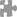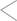# 238008ounf发表于2022-07-27
b-a=c^3+1+3c^2+3c-c^3 =1+3(c^2+c) [1+3(c^2+c)]/3=(c^2+c)……1